## Momentum

MOMENTUM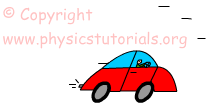Look at the given pictures. If both the car and the truck have same speed, which one can be stopped first? Of course all you say, it is hard to stop truck relative to car. Well, what is the reason making car stop easier? They have same speed but different masses. Can mass effect the stopping time or distance? The answer is again YES! It is hard to stop heavier

objects. What we are talking about so far is momentum.

Momentum is a physical concept that is defined as “moving body”. In other words for talking about momentum we must have moving object, it must have both mass and velocity. Let me formulize what we said;

Momentum=Mass X Velocity

We show momentum in physics with “p”, mass with “m” and velocity with “v”. Then equation becomes;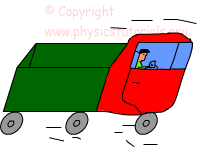p=m.v

Since velocity is a vector quantity and multiplied with mass (scalar quantity) momentum becomes also vector quantity. It has both magnitude and direction. Direction of momentum is the same as velocity. From the definition and given equation we can change momentum by changing its mass or changing its velocity.

Unit of the momentum is kg.m/s as you can guess from the equation.

Example: Calculate the momentum of the give objects.

• A basketball ball having 2kg mass and 6m/s velocity moves to the east
• A car having 15m/s velocity and 1500kg mass moves to the north
• A child having mass 25kg and velocity 2m/s moves to the west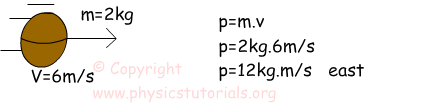b. Momentum of car;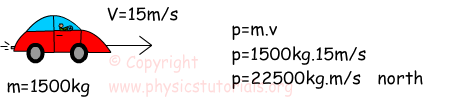c. Momentum of boy;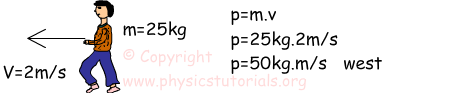Impulse Momentum Exams and Solutions

Author: